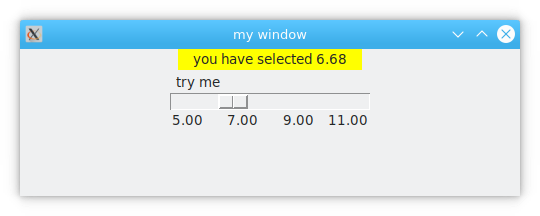# tkinter scale

A tkinter scale lets you select between a range of values. In this article you will learn how to use the tkinter scale with Python

## tkinter scale

To create a scale, use tkinter widget `tk.Scale(master, options)`. Where the parameter master is the parent window. Options are optional options.

``````import tkinter as tk

window = tk.Tk()
window.title('my window')
window.geometry('500x500')

l = tk.Label(window, bg='yellow', width=20, text='empty')
l.pack()

def print_selection(v):
l.config(text='you have selected ' + v)

s = tk.Scale(window, label='try me', from_=5, to=11, orient=tk.HORIZONTAL,
length=200, showvalue=0, tickinterval=2, resolution=0.01, command=print_selection)

s.pack()

window.mainloop()``````## Explanation

Create Windows with the lines below:

``````window = tk.Tk()
window.title('my window')
window.geometry('500x500')``````

Create a display label, a `tk.Label`. As text parameter it takes a `tk.StringVar()`.

``````var1 = tk.StringVar()
l = tk.Label(window, bg='yellow', width=4, textvariable=var1)
l.pack()``````

Refresh window cyclically

``window.mainloop() # Keep refreshing the main window.``

display function

``````def print_selection(v):
l.config(text='you have selected ' + v)``````

Show Scale Control

``````# Note.
# The length is not the width of the character but the width of the pixel.
# showvalue Indicates if the current number is displayed above the horizontal axis, 0 means no display, 1 means display.
# tickinterval tag's unit length 5-7-9-11
# resolution means precision, 0.01 means two decimal places are reserved.
# command indicates the function called, the default value is the value labeled with scale.

s = tk.Scale(window, label='try me', from_= 5, to=11, orient=tk.HORIZONTAL,
length=200, showvalue=0, tickinterval=2, resolution=0.01, command=print_selection)
s.pack()``````